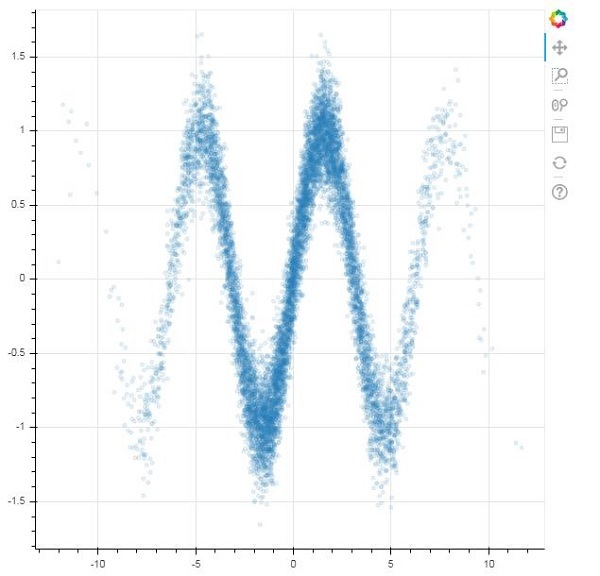# Bokeh - WebGL

When one has to use large datasets for creating visualizations with the help of Bokeh, the interaction can be very slow. For that purpose, one can enable Web Graphics Library (WebGL) support.

WebGL is a JavaScript API that renders content in the browser using GPU (graphics processing unit). This standardized plugin is available in all modern browsers.

To enable WebGL, all you have to do is set output_backend property of Bokeh Figure object to ‘webgl’.

```fig = figure(output_backend="webgl")
```

In the following example, we plot a scatter glyph consisting of 10,000 points with the help of WebGL support.

```import numpy as np
from bokeh.plotting import figure, show, output_file
N = 10000
x = np.random.normal(0, np.pi, N)
y = np.sin(x) + np.random.normal(0, 0.2, N)
output_file("scatterWebGL.html")
p = figure(output_backend="webgl")
p.scatter(x, y, alpha=0.1)
show(p)
```

## Output